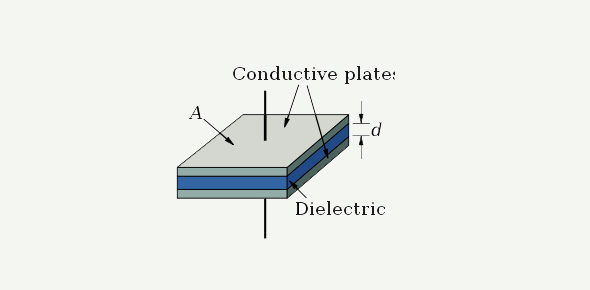# Gibilisco - Capacitance

20 Questions | Total Attempts: 438Settings• 1.
Capacitance acts to store electrical energy as
• A.

Current.

• B.

Voltage.

• C.

A magnetic field.

• D.

An electric field.

• 2.
As capacitor plate area increases, all other things being equal,
• A.

The capacitance increases.

• B.

The capacitance decreases.

• C.

The capacitance does not change.

• D.

The current-handling ability decreases.

• 3.
As the spacing between plates in a capacitor is made smaller, all other things being equal,
• A.

The capacitance increases.

• B.

The capacitance decreases.

• C.

The capacitance does not change.

• D.

The resistance increases.

• 4.
A material with a high dielectric constant
• A.

Acts to increase capacitance per unit volume.

• B.

Acts to decrease capacitance per unit volume.

• C.

Has no effect on capacitance.

• D.

Causes a capacitor to become polarized.

• 5.
A capacitance of 100 pF is the same as which of the following?
• A.

0.01 µF

• B.

0.001 µF

• C.

0.0001 µF

• D.

0.00001 µF

• 6.
A capacitance of 0.033 µF is the same as which of the following?
• A.

33 pF

• B.

330 pF

• C.

3300 pF

• D.

33,000 pF

• 7.
If five 0.050-µF capacitors are connected in parallel, what is the net capacitance of thecombination?
• A.

0.010 µF

• B.

0.25 µF

• C.

0.50 µF

• D.

0.025 µF

• 8.
If five 0.050-µF capacitors are connected in series, what is the net capacitance of thecombination?
• A.

0.010 µF

• B.

0.25 µF

• C.

0.50 µF

• D.

0.025 µF

• 9.
Suppose that two capacitors are connected in series, and their values are 47 pF and 33 pF.What is the net capacitance of this combination?
• A.

80 pF

• B.

47 pF

• C.

33 pF

• D.

19 pF

• 10.
Suppose that two capacitors are in parallel. Their values are 47.0 pF and 470 µF. What is thenet capacitance of this combination?
• A.

47.0 pF

• B.

517 pF

• C.

517 µF

• D.

470 µF

• 11.
Suppose that three capacitors are in parallel. Their values are 0.0200 µF, 0.0500 µF, and0.10000 µF. What is the net capacitance of this combination?
• A.

0.0125 µF

• B.

0.1700 µF

• C.

0.1000 µF

• D.

0.1250 µF

• 12.
The main advantage of air as a dielectric material for capacitors is the fact that it
• A.

Has a high dielectric constant.

• B.

Is not physically dense.

• C.

Has low loss.

• D.

Allows for large capacitance in a small volume.

• 13.
Which of the following is not a characteristic of mica capacitors?
• A.

Excellent efficiency

• B.

Small size, even for large values of capacitance

• C.

High voltage-handling capacity

• D.

Low loss

• 14.
Which of the following capacitance values is most typical of a disk-ceramic capacitor?(a) 100 pF
• A.

100 pF

• B.

33 µF

• C.

470 µF

• D.

10,000 µF

• 15.
Which of the following capacitance values is most typical of a paper capacitor?
• A.

0.001 pF

• B.

0.01 µF

• C.

100 µF

• D.

3300 µF

• 16.
Which of the following capacitance ranges is most typical of an air-variable capacitor?
• A.

0.01 µF to 1 µF

• B.

1 µF to 100 µF

• C.

1 pF to 100 pF

• D.

0.001 pF to 0.1 pF

• 17.
Which of the following types of capacitors is polarized?
• A.

Paper

• B.

Mica

• C.

Interelectrode

• D.

Electrolytic

• 18.
If a capacitor has a negative temperature coefficient, then
• A.

Its capacitance decreases as the temperature rises.

• B.

Its capacitance increases as the temperature rises.

• C.

Its capacitance does not change with temperature.

• D.

It will not work if the temperature is below freezing.

• 19.
Suppose that a capacitor is rated at 33 pF 10%. Which of the following actual capacitancevalues is outside the acceptable range?
• A.

30 pF

• B.

37 pF

• C.

35 pF

• D.

31 pF

• 20.
Suppose that a capacitor, rated at 330 pF, shows an actual value of 317 pF. By how manypercent does its actual capacitance differ from its rated capacitance?
• A.

−0.039%

• B.

−3.9%

• C.

−0.041%

• D.

−4.1%

Related TopicsBack to top